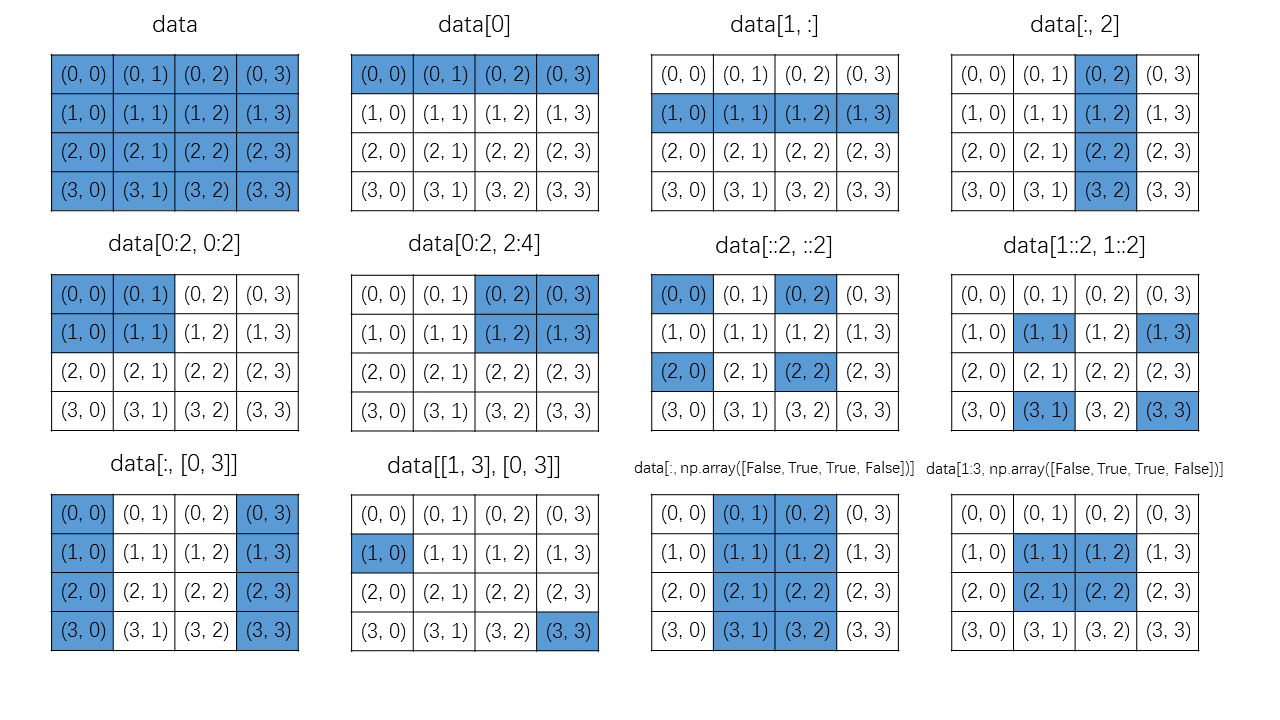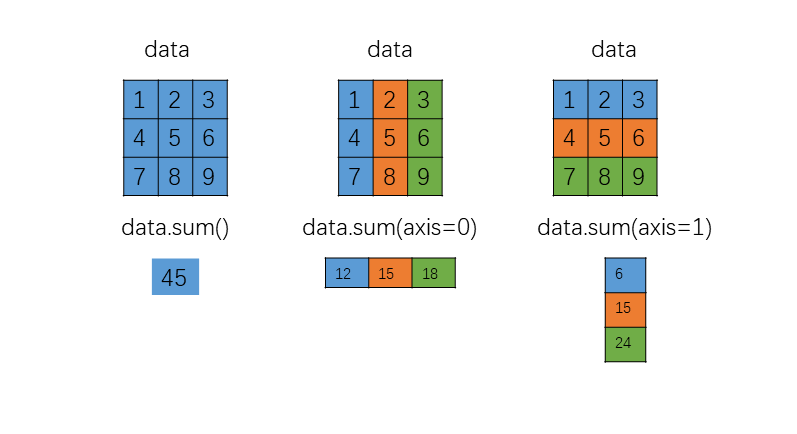NumPy 是 Python 进行数值计算的核心库。在这里，我们介绍了 NumPy 的 n 维数组数据结构：ndarray 对象，并且讨论用于创建和操作数组的函数，包括从数组中提取元素的索引和切片。还讨论了使用 ndarray 对象执行计算的函数和运算符，重点介绍了向量化表达式和运算符，以便使用数组进行高效计算。

## NumPy 数组对象

NumPy 中多维数组的主要数据结构是 ndarray 类。除了存储在数组中的数据之外，该数据结构还包含有关数组的重要元数据，例如其形状、大小、数据类型以及其他属性。有关这些属性的更详细说明，请参阅下表。ndarray docstring 中提供了具有描述的完整属性列表，在 Python 解释器中通过 np.ndarray 调出；在 IPython 控制台通过 np.ndarray? 调出。

shape 元组，值为数组中每一维（轴）的元素个数（长度）
size 数组中元素的总数
ndim 维（轴）数
nbytes 存储数据所需的字节数
dtype 数组中元素的数据类型

### 数据类型

dtype 变体 描述
int int8, int16, int32, int64 整数类型
uint uint8, uint16, uint32, uint64 无符号（非负）整型
bool bool 布尔类型（True False）
float float16, float32, float64, float128 浮点型
complex complex64, complex128, complex256 复数浮点型

NumPy 数组一旦创建其 dtype 就不能改变，但可以使用 type-casted 创建一个拷贝（也可以使用 ndarrayastype 属性）。

## 创建数组

np.array 创建一个数组，其中元素由一个类数组（array-like）对象给出，例如，它可以是 Python 列表、元组、可迭代序列或另一个 ndarray 实例。
np.zeros 创建一个指定维度和数据类型的数组，并将其填充为0
np.ones 创建一个指定维度和数据类型的数组，并将其填充为1
np.diag 创建一个对角阵，指定对角线上的值，并将其他地方填充为0
np.arange 指定开始值、结束值和增量，创建一个具有均匀间隔数值的数组
np.linspace 使用指定数量的元素，在指定的开始值和结束值之间创建一个具有均匀间隔数值的数组
np.logspace 指定开始值和结束值，创建一个具有均匀对数间隔值的数组
np.meshgrid 使用一维坐标向量生成坐标矩阵（和更高维坐标数组）
np.fromfunction 创建一个数组，并用给定函数的函数值填充
np.fromfile 创建一个数组，其数据来自二进制（或文本）文件，NumPy 还提供相应的将 NumPy 数组存储在硬盘中的函数 np.tofile
np.genfromtxt, np.loadtxt 创建一个数组，其数据读取子文本文件，如 CSV 文件，np.genfromtxt 也支持处理缺失值
np.random.rand 创建一个数组，其值在 (0, 1) 之间均匀分布

## 索引与切片

NumPy 数组的元素和子数组可以使用标准的方括号表示法来访问，该表示法也用于 Python 列表。在方括号内，各种不同的索引格式用于不同类型的元素选择。通常，括号内的表达式是一个元组，元组中每一项都指定了数组中相应维（轴）需要选择哪些元素。

### 一维数组

a[m] 选择索引为 m 的元素，其中 m 为整数
a[-m] 从列表末尾开始选择第 m 个元素，其中m是整数
a[m: n] 选择索引从 m 开始到 n-1 结束的元素
a[:], a[0: -1] 选择给定维的所有元素
a[:n] 选择索引从 0 开始 n-1 结束的元素
a[m:], a[m: -1] 选择索引从 m 开始到数组最后的所有元素
a[m: n: p] 选择索引从 m 开始 n 结束，增量为 p 的所有元素
a[: : -1] 以逆序，选择所有元素

### 花式索引与布尔索引## Reshaping 与 Resizing

np.reshape, np.ndarray.reshape 重排一个 N 维数组。元素的总数必须保持不变。
np.ndarray.flatten 创建一个 N 维数组的副本并将其重新解释为一维数组。
np.ravel, np.ndarray.ravel 创建一个 N 维数组的视图（如果可行，否则为副本），在该数组中将其解释为一维数组。
np.squeeze 移除长度为 1 的轴。
np.expand_dims, np.newaxis 向数组中添加长度为 1 的新轴，其中 np.newaxis 用于数组索引。
np.transpose, np.ndarray.transpose, np.ndarray.T 转置数组。
np.hstack 将一组数组水平堆栈（沿着轴 1）。
np.vstack 将一组数组垂直堆栈（沿着轴 0）。
np.dstack 深度（depth-wise）堆栈数组（沿着轴 2）。
np.concatenate 沿着给定轴堆栈数组。
np.resize 调整数组的大小。用给定的大小创建原数组的新副本。如有必要，将重复原数组以填充新数组。
np.append 将新元素添加到数组中。创建数组的新副本。
np.insert 在指定位置将元素插入新数组。创建数组的新副本。
np.delete 删除数组指定位置的元素。创建数组的新副本。

np.ravel（及其相应的 ndarray 方法）是重排的一种特殊情况，它会折叠数组的所有维并返回扁平的一维数组，其长度与原始数组中的元素总数相对应。ndarray 的方法 flatten 具有相同的功能，但返回的是副本而不是视图。

NumPy数组创建后，其元素的数量不能更改。使用函数 np.append、np.insert 和 np.delete 来插入、添加或移除元素，必须创建一个新数组并将数据复制到该数组中。但是由于创建新数组并复制数据的开销，通常需要给数组预分配大小。

## 向量化表达式

### 算术运算

+, +=
--=
**=
/, /=
//, //= 整除
**, **= 指数

### 逐元素操作（element-wise）函数

NumPy 提供向量化函数，对许多基本数学函数和运算进行逐元素地评估。下表给出了 NumPy 中的基本数学函数的总结。这些函数都将单个数组（任意维）作为输入，在对应元素上应用相应的函数，并返回一个具有相同形状的新数组。输出数组的数据类型不一定与输入数组的数据类型相同。

NumPy 函数 描述
np.cos, np.sin, np.tan 三角函数
np.arccos, np.arcsin. np.arctan 反三角函数
np.cosh, np.sinh, np.tanh 双曲三角函数
np.arccosh, np.arcsinh, np.arctanh 反双曲三角函数
np.sqrt 平方根
np.exp 指数
np.log, np.log2, np.log10 底为 e、2 和 10 的对数

NumPy 函数 描述
np.add, np.subtract, np.multiply, np.divide 两个 NumPy 数组的加法、减法、乘法和除法
np.power 将第一个输入参数当作为第二个输入参数的幂（逐元素地）
np.remainder 除法的余数
np.reciprocal 每个元素的倒数
np.real, np.imag, np.conj 输入数组元素的实部、虚部和复共轭
np.sign, np.abs 符号值和绝对值
np.floor, np.ceil, np.rint 转换为整数
np.round 舍入到给定的小数点位数

### 聚合函数

NumPy 提供了一组用于计算数组的聚合函数，它们将数组作为输入并将标量作为默认输出返回。诸如计算输入数组中元素的均值、标准偏差和方差以及计算数组中元素的总和和乘积的函数都是聚合函数。

NumPy 函数 描述
np.mean 数组中所有值的均值
np.std 标准偏差
np.var 方差
np.sum 所有元素的总和
np.prod 所有元素的乘积
np.cumsum 所有元素的累积和
np.cumprod 所有元素的累积乘
np.min, np.max 数组中的最小/最大值
np.argmin, np.argmax 数组中最小/最大值的索引
np.all 如果参数数组中的所有元素都不为零，则返回 True
np.any 如果参数数组中的任何元素不为零，则返回 True### 布尔数组与条件表达式

NumPy 数组可以与常见的比较运算符一起使用。广播规则也适用于比较运算符，如果两个数组形状兼容，则返回布尔数组。

np.where 根据条件数组的值从两个数组中选择值
np.choose 根据给定索引数组的值从数组列表中选择值
np.select 根据条件列表从数组列表中选择值
np.nonzero 返回非零元素的索引
np.logical_and 逐元素地执行 AND 操作
np.logical_or, np.logical_xor 逐元素地执行 OR/XOR 操作
np.logical_not 逐元素地执行 NOT 操作

np.select 函数的工作原理与此类似，但它的参数是布尔条件数组的列表及相应的值数组的列表：

np.choose 将列表或数组作为第一个参数，该列表或数组的索引决定了从给定数组列表中的哪个数组中选取元素：

### 集合运算

Python 语言为管理无序唯一对象集提供了便利的 set（集合） 数据结构。NumPy 数组的类 ndarray 也可用于描述这样的集合。NumPy 提供的用于集合运算的函数可总结为下表。

np.unique 使用唯一元素创建新数组，其中每个值只出现一次
np.in1d 数组中元素是否存在另一数组中
np.intersect1d 返回给定的两个数组中都包含的元素
np.setdiff1d 返回给定的两个数组中一个数组包含而另一个数组不包含的元素
np.union1d 返回给定的两个数组的所有元素

### 数组上的运算

np.transpose, np.ndarray.transpose, np.ndarray.T 数组的转置
np.fliplr/np.flipud 反转每行/列中的元素
np.rot90 将前两个轴上的元素旋转 90 度
np.sort, np.ndarray.sort 对指定轴（默认为数组最后一个轴）的元素进行排序 np.ndarray 方法执行排序，修改输入数组

## 矩阵与向量运算

np.dot 表示向量、数组或张量的两个给定数组之间的矩阵乘法（点乘）
np.inner 表示向量的两个数组之间的标量积（内积）
np.cross 表示向量的两个数组之间的叉积
np.tensordot 沿着多维数组的指定轴点乘
np.outer 表示向量的两个数组之间的外积（向量的张量积）
np.kron 表示矩阵和高维数组的数组之间的 Kronecker 乘积（矩阵的张量积）
np.einsum 评估多维数组的爱因斯坦求和约定（Einstein summation convention）

np.asmatrix 函数以 np.matrix 实例的形式创建原始数组的视图。